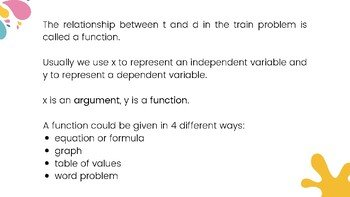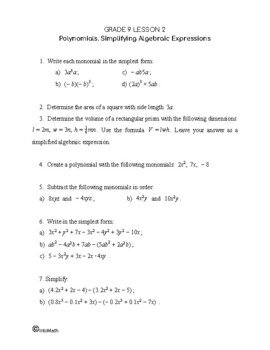CA$2.00 This lesson builds up on the previous one and takes it further. You will discover a linear function and its meaning. You will also find out how to represent a linear function in 4 different ways: equation, table of values, graph and words. You will learn about two types of linear functions: one that passes through the origin when graphed and the other one that doesn’t. This lesson will explain what each letter stands for in the equation y = mx + b. When the relationship is linear, the rate of change is constant. We have already observed it in the previous lesson. The relationship between distance and time is a great example of a linear function. When graphed it produces a straight line.Grade 9 Workbook (14 pages with answers) CA$4.00

The slope of a line represents the rate of change of one quantity in relation to the other. It can be calculated using any two points that sit on the line. Since each point has the coordinates (ordered pair), the slope of a line is calculated by finding the difference between x and y coordinates of the points respectively.

We use letter “m” to represent the slope of a line:

m = (y2 – y1)/(x2 – x1)